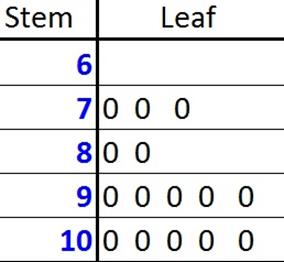© Ms. Garcia Math 7th The stem-and- leaf plot below shows test scores in Math. GR37 answersUse the stem and leaf plot to answer these questions. 1) What is the mean? 2) What is the median? 88 90 Answer Answer 3) What is the mode? 4) What is the range? 90 30 Answer Answer 5) What is the maximum? 6) What is the total number of students tested? 100 15 Answer Answer Name: ID: